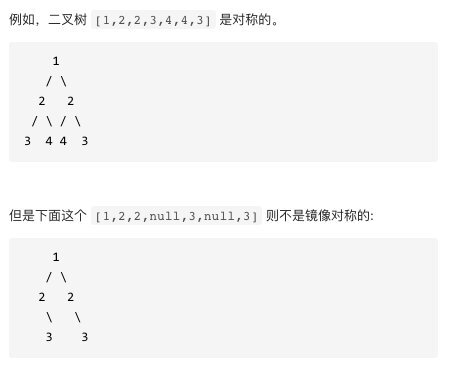# 手撸二叉树之对称二叉树HelloWorld杰少## 题目：

### Example:## 思路分析:

1. 它们的根节点具有相同的值

2. 每个树的右子树都与另一个树的左子树镜像对称

### 递归实现：

/** * Definition for a binary tree node. * public class TreeNode { *     int val; *     TreeNode left; *     TreeNode right; *     TreeNode() {} *     TreeNode(int val) { this.val = val; } *     TreeNode(int val, TreeNode left, TreeNode right) { *         this.val = val; *         this.left = left; *         this.right = right; *     } * } */class Solution {    public boolean isSymmetric(TreeNode root) {        //调用递归函数，比较左节点，右节点        return isSame(root, root);    }    public boolean isSame(TreeNode p, TreeNode q) {        //递归的终止条件是两个节点都为空        if (p == null && q == null) {            return true;        }        //或者两个节点中有一个为空        if (p == null || q == null) {            return false;        }        //两个节点的值相等        //再递归的比较 左节点的左孩子 和 右节点的右孩子    //以及比较  左节点的右孩子 和 右节点的左孩子        return p.val == q.val && isSame(p.left, q.right) && isSame(p.right, q.left);               }}

1. 首先从队列中拿出两个节点(left 和 right)比较

2. 将 left 的 left 节点和 right 的 right 节点放入队列

3. 将 left 的 right 节点和 right 的 left 节点放入队列

### 迭代实现

class Solution {  public boolean isSymmetric(TreeNode root) {    if(root==null || (root.left==null && root.right==null)) {      return true;    }    //用队列保存节点    LinkedList<TreeNode> queue = new LinkedList<TreeNode>();    //将根节点的左右孩子放到队列中    queue.add(root.left);    queue.add(root.right);    while(queue.size()>0) {      //从队列中取出两个节点，再比较这两个节点      TreeNode left = queue.removeFirst();      TreeNode right = queue.removeFirst();      //如果两个节点都为空就继续循环，两者有一个为空就返回false      if(left==null && right==null) {        continue;      }      if(left==null || right==null) {        return false;      }      if(left.val!=right.val) {        return false;      }      //将左节点的左孩子， 右节点的右孩子放入队列      queue.add(left.left);      queue.add(right.right);      //将左节点的右孩子，右节点的左孩子放入队列      queue.add(left.right);      queue.add(right.left);    }        return true;  }}

## 最后## 评论